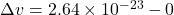Question

) A 6.50 kg rock starting from rest free-falls through a distance of 30.0 m. a. Assuming no air resistance, find the amount of momentum that is transferred from the rock to earth as it collides with earth’s surface in a perfectly inelastic collision. b. What is the change in velocity of earth as a result of this momentum change? The earth’s mass is 5.972 x 10^24 kg. Show all your work, assuming the rock–earth system is closed.

1.Euphemia

##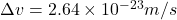Explanation:

Velocity of the stone just before it will strike the Earth is given as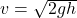so we will have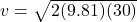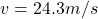Now by momentum conservation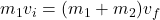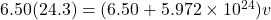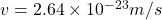Part a)

Momentum transferred by the stone is given as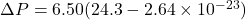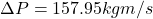Part b)

Change in velocity of Earth